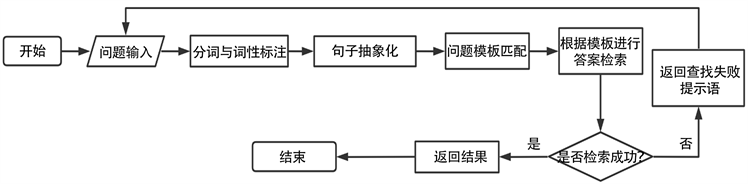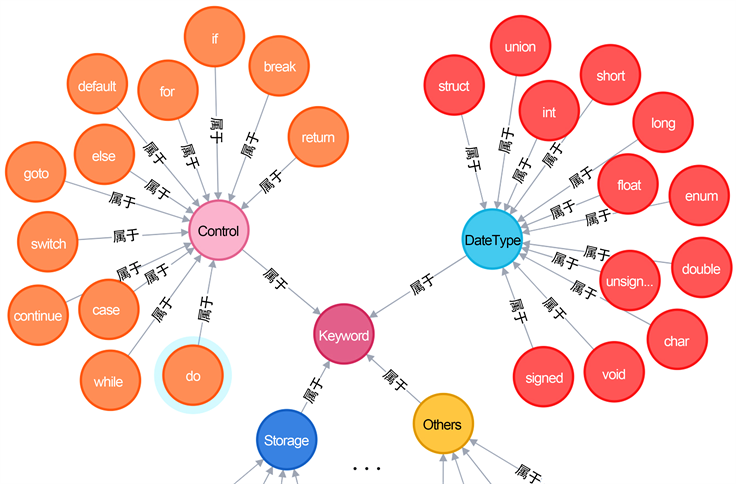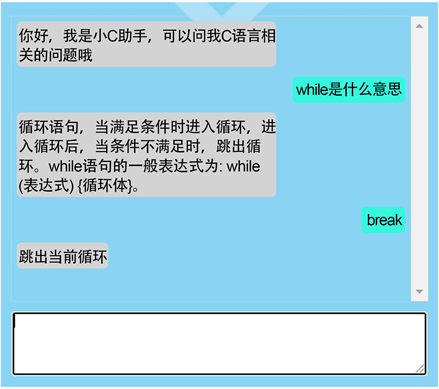#### 期刊菜单

The Design and Implementation of the C Language Question and Answer System Based on the Knowledge Base
DOI: 10.12677/CSA.2021.1112315, PDF , HTML, XML, 下载: 246  浏览: 597  科研立项经费支持

Abstract: Question answer system can provide fast and accurate knowledge acquisition channels. This paper designed a C language question and answer system based on knowledge. First, an ontology knowledge based on a custom dictionary was constructed, and question templates were designed. Then the classifier was used to match questions and the templates. Finally, the result was returned from knowledge. The experimental results show that the system designed in this paper can match the question and answer of C language knowledge.

1. 引言

C语言初学者都会受到语法不清、编译报错的困扰，无从下手。他们获取解决方法途径是利用传统的信息检索，在网上大海捞针般地查找答案。这种方式虽然能够快速地反馈给用户大量的相关信息，但是信息冗余高，有效信息少，实际效率低。

2. 相关工作研究

2.1. 知识图谱

2.2. 知识推理

3. 系统架构Figure 1. Question and answer system flow chart

1) 原始问句：while是什么意思；

2) 分词：while/key是/v什么/r意思/n；

3) 句子抽象化：key是什么意思；

4) 套用问题模板：key简介；

5) 本体替换：while简介；

6) 生成的查询语句：match(n) where n.name Contains while return n.description；

7) 返回结果：循环语句，当满足条件时进入循环，进入循环后，当条件不满足时，跳出循环。while语句的一般表达式为：while(表达式){循环体}。Figure 2. Question and answer processing flow

4. 本体与知识图谱的构建

4.1. 本体及其作用

1) 在爬取问答系统所需的数据时，利用本体可以快速确定网站页面内容是否与本领域相关。

2) 在问句处理过程中，本体与本体之间的相互关系，本体的属性及约束关系都可以为问句预处理提供很好的支持。

4.2. C语言领域本体

4.3. 本体的属性

4.4. 建立知识图谱Figure 3. Construction a knowledge graphFigure 4. Partial knowledge graph

5. 问题模板设计

5.1. 问题模板简介

1) “int在C语言中的作用是什么？”

2) “C语言中的int是啥意思？”

3) “int是干什么用的？”

5.2. 建立问题模板

1) 询问本体的概念；

2) 询问多个本体间的关系；

3) 询问本体的属性；

4) 询问本体的相关操作；Table 1. Part of the question template

5.3. 问题模板匹配原理Figure 5. Naive Bayes classifier workflow

$p\left(Y|X\right)=\frac{p\left(X|Y\right)p\left(Y\right)}{p\left(X\right)}$ (1)

$P\left({y}_{k}|{x}_{1},\cdots ,{x}_{n}\right)=\frac{P\left({x}_{1}|{y}_{k}\right)P\left({x}_{2}|{y}_{k}\right)\cdots P\left({x}_{n}|{y}_{k}\right)P\left({y}_{k}\right)}{P\left({x}_{1}\right)P\left({x}_{2}\right)\cdots P\left({x}_{n}\right)}$ (2)

· ${x}_{1},{x}_{2}\cdots ,{x}_{n}$ 为已经发生的事件，在本系统中它们是句子中被分解出来的每一个特征词；

· ${y}_{k}$ 为一个待求解事件，在本系统中为已知的 $k$ 个问题模板；

· $P\left({x}_{n}|{y}_{k}\right)$ 以及其余的变量则是通过训练集训练得到的已知的概率。

6. 系统实现与结果展示Table 2. Matching accuracy of different templates under the same test volumeFigure 6. System operation renderings

7. 结束语

NOTES

*通讯作者。

  刘园园, 李劲华, 赵俊莉. 基于语义解析的领域问答系统的设计与实现[J]. 计算机应用与软件, 2021, 38(11): 42-48+97.  刘爽, 杨辉, 李佳宜, 谭楠楠. 面向多数据源的少数民族文化知识图谱构建[J]. 计算机技术与发展, 2021, 31(8): 191-197+203.  韩涛, 黄海松, 姚立国. 面向航空发动机故障知识图谱构建的实体抽取[J]. 组合机床与自动化加工技术, 2021(10): 69-73+78.  吴岳忠, 沈雪豪, 肖发龙, 邓芝一, 李长云. 基于知识图谱的包装领域智能问答系统的设计[J]. 包装工程, 2021, 42(15): 203-210.  何政, 叶刚. 基于知识图谱的C#课程学习导航系统研究[J]. 太原城市职业技术学院学报, 2021(9): 97-99.  李家瑞, 李华昱, 闫阳. 面向多源异质数据源的学科知识图谱构建方法[J]. 计算机系统应用, 2021, 30(10): 59-67.  廖子慧. 基于知识图谱的英语语法智能题库系统研建[D]: [硕士学位论文]. 北京: 北京林业大学, 2020.  张紫薇. 基于机器学习的知识推理的研究与设计[D]: [硕士学位论文]. 北京: 北京邮电大学, 2021.  刘焕勇, 薛云志, 李瑞, 任红萍, 陈贺, 张鹏. 面向开放文本的逻辑推理知识抽取与事件影响推理探索[J]. 中文信息学报, 2021, 35(10): 56-63.  李诗轩, 陈烨, 石文萱, 杨达森. 基于知识推理的上市公司财务危机预警研究[J]. 武汉理工大学学报(信息与管理工程版), 2021, 43(4): 322-329.  王峻. 基于属性分组的扩展朴素贝叶斯分类器[J]. 洛阳理工学院学报(自然科学版), 2021, 31(3): 85-88+93.  杨航, 刘赪, 夏美美, 范元静. 基于R-vine Copula理论的改进朴素贝叶斯分类器[J]. 甘肃科学学报, 2021, 33(3): 12-16.  贾宇彤. 基于知识图谱的知识推理研究[D]: [硕士学位论文]. 成都: 电子科技大学, 2021.  Wang, L., Liu, H., Zhou, T., Liang, W. and Shan, M. (2021) Multidimensional Emotion Recognition Based on Semantic Analysis of Biomedical EEG Signal for Knowledge Discovery in Psychological Healthcare. Applied Sciences, 11, Article No. 1338. https://doi.org/10.3390/app11031338  关慧, 吕颖, 贾成真. 基于句法和语义的需求依赖关系自动获取[J]. 计算机技术与发展, 2021, 31(2): 20-26.  Chen, K., Shen, G., Huang, Z. and Wang, H. (2021) Improved Entity Linking for Simple Question Answering Over Knowledge Graph. International Journal of Software Engineering and Knowledge Engineering, 31, 55-80. https://doi.org/10.1142/S0218194021400039